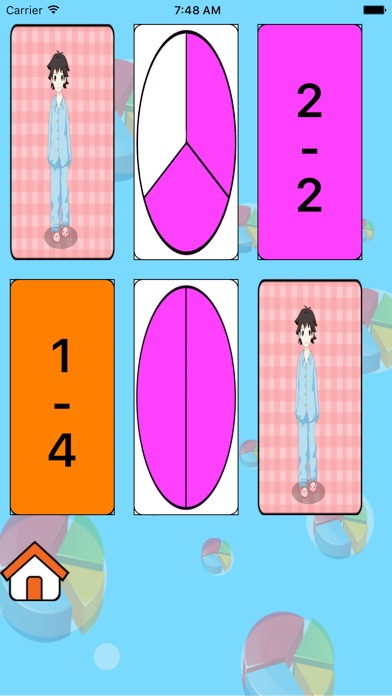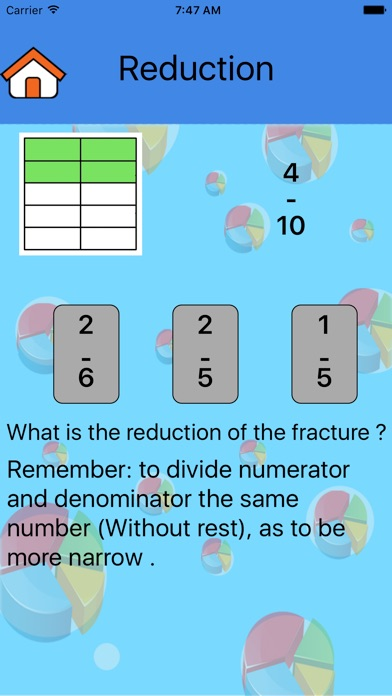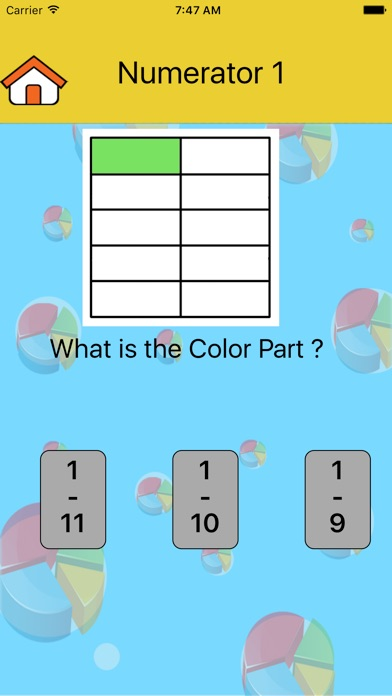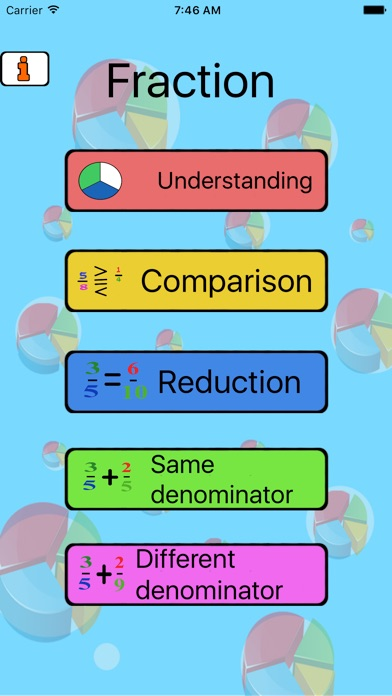# fraction lite

## iOS UniversalEducation

Free

Software for learning fractions:
Some learning math is debris.
How obtained a fragment?
Following the crisis of distribution, ie, operation, division.

Where we run into fractions?
Baking / cooking - a half cup of oil.
Construction - half a bucket of mortar.
School - half from school came ....

The software was built as part of a study of fragments in stages.

1. Understand first phase fault:
1.1 Understand the pieces with numbers 1.
2.1 Understand the debris with variable numbers.
3.1 Linkage memory game broke a number of image.

2. Comparing Fractions
At this time we will learn to identify the relationship between fractions with pictures.

3. Cancellation of fractions
As part of preparation for connecting subtracting fractions with same denominator not
Learn at this stage to identify fragments and their reduction.

4. Same denominator
1.4 Connection of the fragments with the same denominator
2.4 Subtraction of fractions with same denominator

5. Calls not identical
1.5 Connection of the fragments are not identical calls
2.5 Subtraction of fractions with same denominator not
Another practice of decimals

from decimals to fraction

from fraction to decimal

••••